# Identical Particles and Pauli Exclusion Principle - Quantum Mechanics, CSIR-NET Physical Sciences Physics Notes | EduRev

## Physics : Identical Particles and Pauli Exclusion Principle - Quantum Mechanics, CSIR-NET Physical Sciences Physics Notes | EduRev

The document Identical Particles and Pauli Exclusion Principle - Quantum Mechanics, CSIR-NET Physical Sciences Physics Notes | EduRev is a part of the Physics Course Physics for IIT JAM, UGC - NET, CSIR NET.
All you need of Physics at this link: Physics

Introduction

There are many systems in nature that are made of several particles of the same species.
These particles all have the same mass, charge, and spin. For instance the electrons in an atom are identical particles. Identical particles cannot be distinguished by measuring their properties. This is also true for classical particles. In classical mechanics we can always follow the tra jectory of each individual particle, i.e. their time evolution in space. The tra jectories identify each particle in classical mechanics, making identical particles distinguishable.

In quantum mechanics the concept of tra jectory does not exist and identical particles are indistinguishable. Let us consider for simplicity a system of two identical particles. The state of the system is described by a wave function: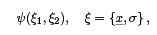(16.1)

where x yields the position of the particle, and σ yields the z-component of the spin of the particle, if the latter is diﬀerent from zero.
The state with the two particles exchanged is described by the wave function: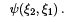(16.2)

If the two particles are identical, the two functions represent the same quantum state, and therefore: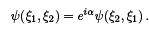(16.3)

Repeating the exchange of the two particles we ﬁnd: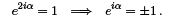(16.4)

Hence the wave function of a system of two identical particles must be either symmetric or antisymmetric under the exchange of the two particles.

The Spin-Statistics Theorem

 Systems of identical particles with integer spin (s =0, 1, 2,. ..), known as bosons ,have wave functions which are symmetric under interchange of any pair of particle labels. The wave function is said to obey Bose-Einstein statistics.Systems of identical particles with half-odd-integer spin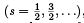, known as fermions , have wave functions which are antisymmetric under interchange of any pair of particle labels. The wave function is said to obey Fermi-Dirac statistics.

This law was discovered by Wolfgang Pauli and is supported by experimental evidence.

A ﬁrst look at Helium

In the simplest model of the helium atom, the Hamiltonian is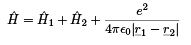where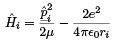Note that it is symmetric under permutation of the indices 1 & 2 which label the two electrons.

This must be the case if the two electrons are identical or indistinguishable : it cannot matter which particle we label 1 and which we label 2.

This observation is quite general: the same argument holds for identical particles other than electrons and can be extended to systems of more than two identical particles.

Let us write the symmetry condition concisely as: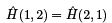Suppose that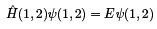then interchanging the labels 1 & 2 gives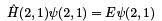but using the symmetry property of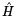means that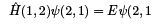so we conclude that ψ(1, 2) and ψ(2, 1) are both eigenfunctions belonging to the same eigenvalue, E , as is any linear combination of ψ(1, 2) and ψ(2, 1). In particular, the normalised symmetric and antisymmetric combinations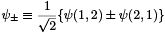are eigenfunctions belonging to the eigenvalue, E .

If we introduce a particle interchange operator, P12 , with the property that

P12ψ(1, 2) = ψ(2, 1)

then the symmetric and antisymmetric combinations are eigenfunctions of  P12  with eigenvalues ±1respectively:

P12ψ± = ±ψ± Since ψ± are simultaneous eigenfunctions ofand P12 it follows that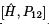= 0, as you can easily verify from the above equations, and that the symmetry of the wavefunction is a constant of the motion.

Two-electron wave function

In the previous lecture, we constructed the states of the coupled representation for two spin- 1/2 electrons, the three triplet states: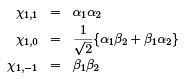and the singlet state: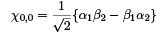where we have used a simpliﬁed notation for the states of the coupled basis: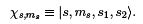Notice that the triplet states are symmetric under interchange of the labels 1 and 2, whereas the singlet state is antisymmetric . If we are to satisfy the Spin-Statistics Theorem, this has implications for the symmetry of the spatial wavefunctions that we combine with the spin functions to give the full wavefunction of the 2-electron system. The 2-electron wavefunction will have the general form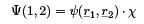The symmetry properties of the various factors are as follows: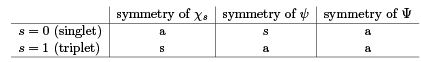Thus the spatial wavefunction must be antisymmetric if the two electrons are in a spin triplet state but symmetric if they are in a spin singlet state.

More on the He atom

Suppose for the moment that we neglect spin and also neglect the mutual Coulomb repulsion between the two electrons. That is, we treat the two electrons as moving independently in the Coulomb ﬁeld of the nucleus. The Hamiltonian then reduces to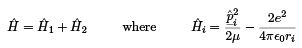We already know what the eigenfunctions and eigenvalues for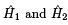are, namely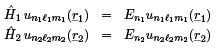so it is easy to see thathas eigenfunctions which are just products of the 1-electron eigenfunctions: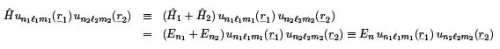Thus the energy eigenvalues are given by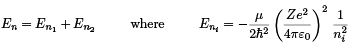The Ground State:

In this crude model the ground state energy is just

En=1 = En1=1 + En2=1 =2 En1=1.

Setting Z = 2 in the Bohr formula thus yields for the ground state energy: E1 =8 × (−13.6 eV )= −108.8 eV to be compared with the experimentally measured value of −78.957 eV .

The ground state spatial wavefunction has n= n2 = 1 and ℓ1 = ℓ= m= m2 = 0 and is thus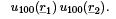Each electron is in a 1s state; we say that the electronic conﬁguration is (1s)2 .
If we now worry about spin, we remember that the total wavefunction is a product of a spatial wavefunction and a spin wavefunction of the correct symmetry. But the spatial wavefunction is symmetric and can thus only be combined with a spin singlet spin function to give an overall antisymmetric 2-electron wavefunction;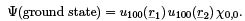Notice that, since ℓ1 = ℓ2 = 0, the total orbital angular momentum quantum number of the ground state conﬁguration is ℓ = ℓ1 + ℓ2 = 0. Thus the ground state has zero orbital and spin angular momentum, and hence zero total angular momentum.

The First Excited States:

The ﬁrst excited states correspond to one electron being excited to a 2s or 2p state, with the other remaining in a 1s state. The electronic conﬁgurations are denoted by (1s)(2s) and (1s)(2p) respectively. The (degenerate) energy eigenvalue can again be obtained from the Bohr formula with Z = 2: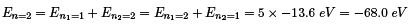In this case it is possible to construct spatial wavefunctions which are either symmetric or antisymmetric . The overall antisymmetric combinations are then: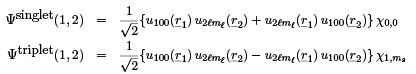Pauli exclusion principle

The results that we have just obtained for the independent particle approximation to the helium atom illustrate a more general result, related to the Spin-Statistics Theorem and known as the Pauli Exclusion Principle which states

 No two identical fermions can be in the same quantum state

For example,

• in the ground state, we see that although both electrons have n = 1 and ℓ = m =0 i.e. both are in a 1s state, they are in a spin singlet state, which means that if one electron is in the spin state α, the other must be in the state β : the two electrons cannot have an identical set of quantum numbers; if both were in the spin state α, the 2-electron spin state would be a triplet state, which is ruled out by the Spin-Statistics Theorem;
• in any excited state, both electrons can be in the spin state α, corresponding to the triplet state, but then the spatial wavefunction is forced to be antisymmetric, so that the quantum numbers n, ℓ , m , of the two electrons have to diﬀer - otherwise the spatial wavefunction would vanish identically!

No such restriction applies to identical bosons; any number of identical bosons can occupy the same quantum state.

Note that the correlation between spin and statistics has been postulated in the nonrelativistic context used inthis course. The spin-statistic theorem can actually be derived in a relativistic formulation of quantum mechanics. It is a consequence of the principles of special relativity, quantum mechanics, and the positivity of the energy.

Offer running on EduRev: Apply code STAYHOME200 to get INR 200 off on our premium plan EduRev Infinity!

159 docs

,

,

,

,

,

,

,

,

,

,

,

,

,

,

,

,

,

,

,

,

,

,

,

,

;